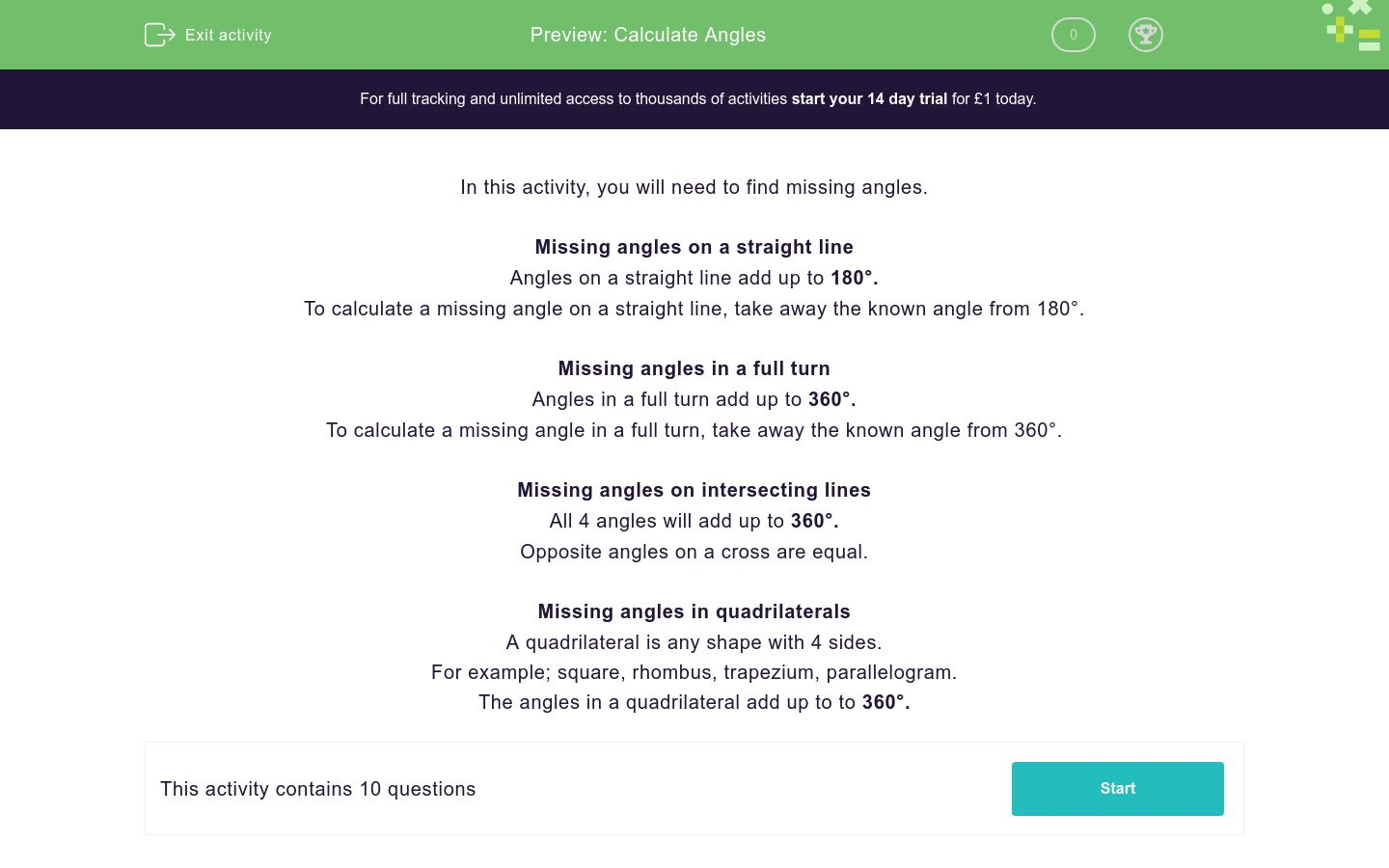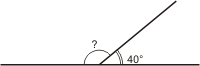# Calculate Angles

In this worksheet, students will be asked to calculate the missing angles on a straight line, in a quadrilateral, around a point and on an intersecting line.Key stage:  KS 2

Curriculum topic:   Geometry: Properties of Shapes

Curriculum subtopic:   Recognise/Find Angles

Difficulty level:### QUESTION 1 of 10

In this activity, you will need to find missing angles.

Missing angles on a straight line

Angles on a straight line add up to 180°.

To calculate a missing angle on a straight line, take away the known angle from 180°.

Missing angles in a full turn

Angles in a full turn add up to 360°.

To calculate a missing angle in a full turn, take away the known angle from 360°.

Missing angles on intersecting lines

All 4 angles will add up to 360°.

Opposite angles on a cross are equal.

A quadrilateral is any shape with 4 sides.

For example; square, rhombus, trapezium, parallelogram.

Look at the diagram below.

What is the missing angle?112°

130°

140°

120°

Look at the diagram below.

What is the missing angle?

Write the correct answer (just write the number)Look at the diagram below.

What is the missing angle?

Write the correct answer (just write the number)Can you choose the correct answer for the missing angle 'x'?84°

80°

85°

90°

Can you choose the correct answer for the missing angle 'x'?116°

106°

126°

96°

Can you write the correct answer for the missing angle 'x'

Just write the number.Look at the diagram below that shows the angles around a point.

Can you choose the correct number from the grid for the missing angle?Look at the diagram below that shows the angles around a point.

Can you write the correct number for the missing angle?

Just write the number.Look at the diagram below showing intersecting lines.

The angle opposite 65° will also be 65° as opposite angles are equal.

We know that the angles around a point will have to total 360°.

Can you calculate what one of the other missing angles will be worth?

(Remember they will both be worth the same as they are opposite).

Choose from the options below.115°

105°

125°

135°

Look at the diagram below showing intersecting lines.

The angle opposite 85° will also be 85° as opposite angles are equal.

We know that the angles around a point will have to total 360°.

Can you calculate what one of the other missing angles will be worth?

(Remember they will both be worth the same as they are opposite).90°

95°

85°

75°

• Question 1

Look at the diagram below.

What is the missing angle?120°
EDDIE SAYS
Remember the angles in a straight line add up to 180°. Therefore 180 - 60 = 120°.
• Question 2

Look at the diagram below.

What is the missing angle?

Write the correct answer (just write the number)115
EDDIE SAYS
Again, remember the rule that the angles in a straight line add up to 180°. Therefore 180 - 65 = 115°.
• Question 3

Look at the diagram below.

What is the missing angle?

Write the correct answer (just write the number)EDDIE SAYS
Again, remember the rule that the angles in a straight line add up to 180°. Therefore 180 - 40 =140°. Don’t panic if you found this difficult, we'll keep practising.
• Question 4

Can you choose the correct answer for the missing angle 'x'?85°
EDDIE SAYS
Remember, the angles in a quadrilateral add up to a total of 360°. The first step was to add all of the angles you knew about together: 126° + 77° + 72 ° = 27° Then subtract this from 360° 360° - 275° = 85°
• Question 5

Can you choose the correct answer for the missing angle 'x'?116°
EDDIE SAYS
Remember the angles in a quadrilateral add up to a total of 360°. Did you spot that this quadrilateral had a right angle worth 90°? The first step was to add all of the angles you knew about together: 98° + 90° + 56° = 244° Then subtract this from 360° 360° - 244° = 116°
• Question 6

Can you write the correct answer for the missing angle 'x'

Just write the number.82
EDDIE SAYS
Remember the angles in a quadrilateral add up to a total of 360°. Again, did you spot that this quadrilateral has got a right angle worth 90°? The first step was to add all of the angles you knew about together: 129° + 90° + 59 ° = 278 Then subtract this from 360° 360° - 278° = 82° Is this starting to get easier for you?
• Question 7

Look at the diagram below that shows the angles around a point.

Can you choose the correct number from the grid for the missing angle?EDDIE SAYS
To tackle this one, the rule to remember is that angles around a point total 360°. Firstly, add all of the angles you have been given together 105 + 90 + 25 = 220° 360 - 220 = 140°
• Question 8

Look at the diagram below that shows the angles around a point.

Can you write the correct number for the missing angle?

Just write the number.EDDIE SAYS
Again, we needed the rule that the angles around a point total 360°. As we have only been given one angle, we subtract that directly from 360° 360° - 75° = 285°
• Question 9

Look at the diagram below showing intersecting lines.

The angle opposite 65° will also be 65° as opposite angles are equal.

We know that the angles around a point will have to total 360°.

Can you calculate what one of the other missing angles will be worth?

(Remember they will both be worth the same as they are opposite).

Choose from the options below.115°
EDDIE SAYS
The first step was to multiply 65° by 2 = 130°. Next 360° - 130° = 230° 230° is the total for the 2 missing angles. We then had to divide 230° by 2 to find what that one missing angle is worth. 230° ÷ 2 = 115°.
• Question 10

Look at the diagram below showing intersecting lines.

The angle opposite 85° will also be 85° as opposite angles are equal.

We know that the angles around a point will have to total 360°.

Can you calculate what one of the other missing angles will be worth?

(Remember they will both be worth the same as they are opposite).95°
EDDIE SAYS
The first step was to multiply 85° x 2 = 170°. Next, 360° - 170° = 190° 190° is the total for the 2 missing angles. We then had to divide 190° by 2 to find that one missing angle is worth. 190° ÷ 2 = 95° Practise makes perfect, that’s another activity completed!
---- OR ----

Sign up for a £1 trial so you can track and measure your child's progress on this activity.

### What is EdPlace?

We're your National Curriculum aligned online education content provider helping each child succeed in English, maths and science from year 1 to GCSE. With an EdPlace account you’ll be able to track and measure progress, helping each child achieve their best. We build confidence and attainment by personalising each child’s learning at a level that suits them.

Get started